# Class_1._Introduction.pptx

Mar. 25, 2023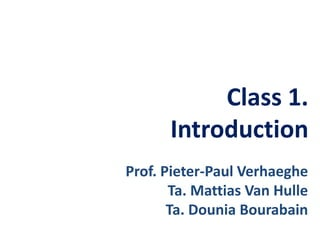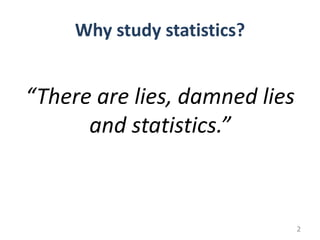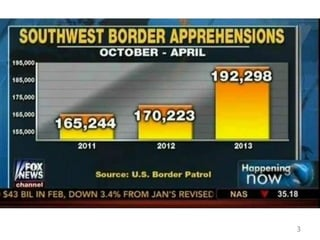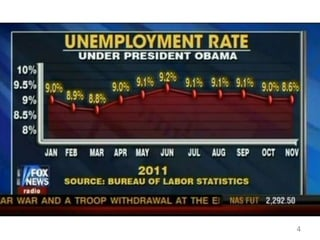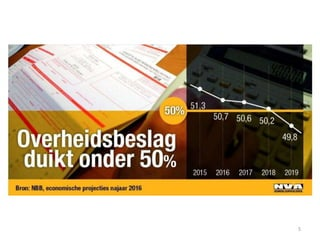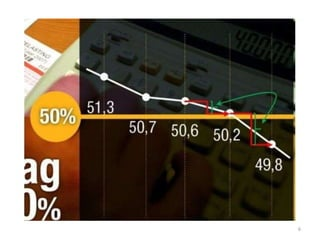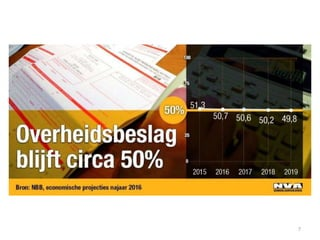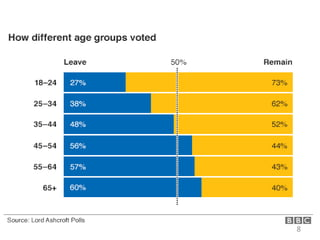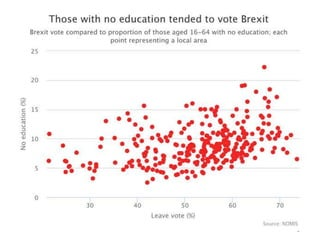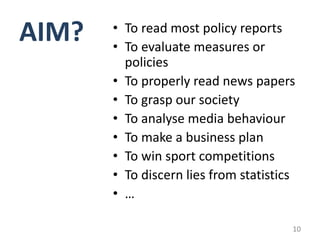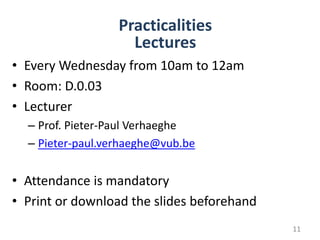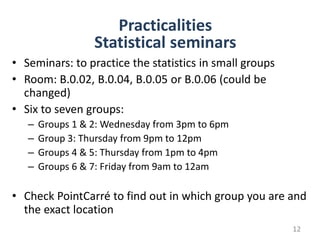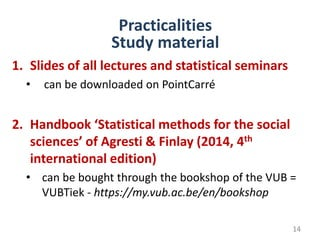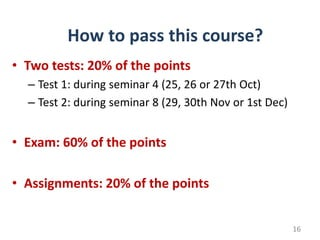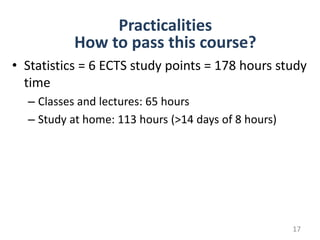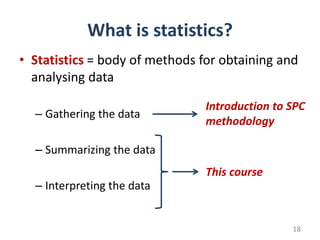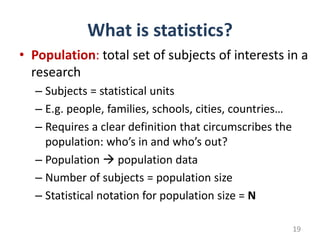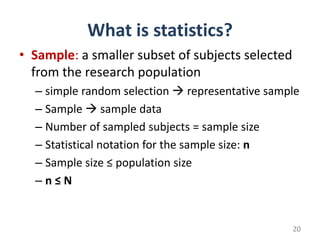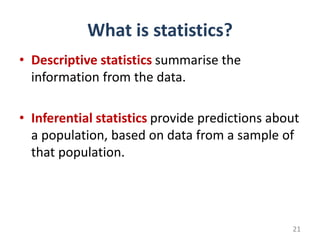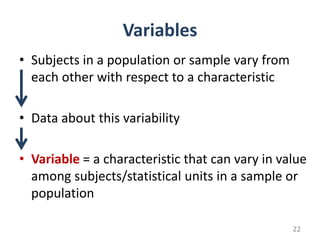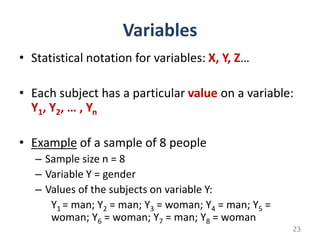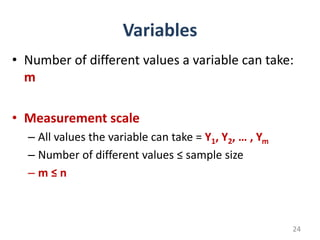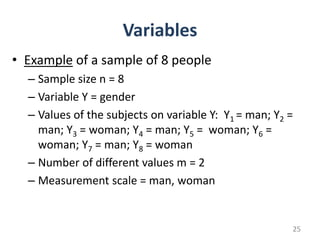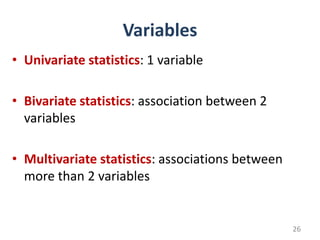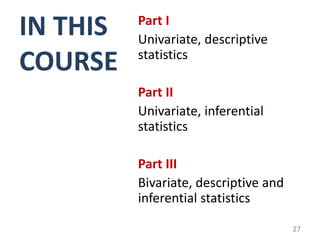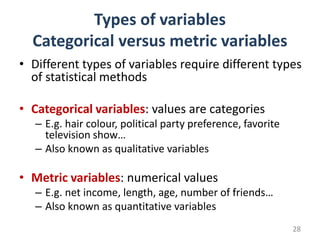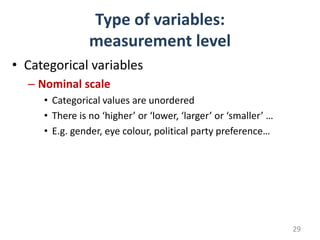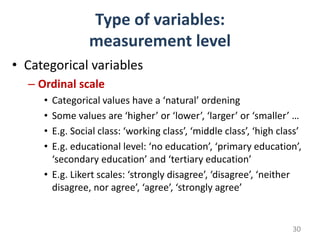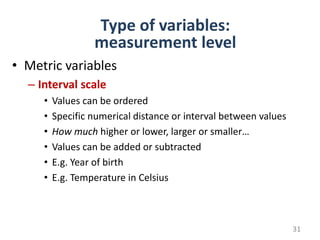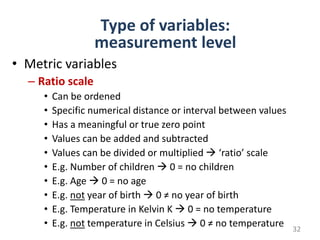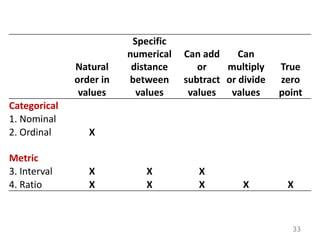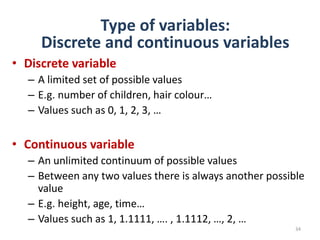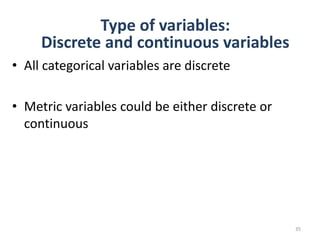1 of 36

### Class_1._Introduction.pptx

1. Class 1. Introduction Prof. Pieter-Paul Verhaeghe Ta. Mattias Van Hulle Ta. Dounia Bourabain
2. Why study statistics? “There are lies, damned lies and statistics.” 2
3. 3
4. 4
5. 5
6. 6
7. 7
8. 8
9. 9
10. AIM? • To read most policy reports • To evaluate measures or policies • To properly read news papers • To grasp our society • To analyse media behaviour • To make a business plan • To win sport competitions • To discern lies from statistics • … 10
11. • Every Wednesday from 10am to 12am • Room: D.0.03 • Lecturer – Prof. Pieter-Paul Verhaeghe – Pieter-paul.verhaeghe@vub.be • Attendance is mandatory • Print or download the slides beforehand Practicalities Lectures 11
12. • Seminars: to practice the statistics in small groups • Room: B.0.02, B.0.04, B.0.05 or B.0.06 (could be changed) • Six to seven groups: – Groups 1 & 2: Wednesday from 3pm to 6pm – Group 3: Thursday from 9pm to 12pm – Groups 4 & 5: Thursday from 1pm to 4pm – Groups 6 & 7: Friday from 9am to 12am • Check PointCarré to find out in which group you are and the exact location Practicalities Statistical seminars 12
13. Mattias Van Hulle TA for Groups 1 & 4 & 6 Mattias.van.hulle@vub.be Practicalities Teaching assistants 13 Dounia Bourabain TA for Groups 2 & 3 & 5 & 7 Dounia.bourabain@vub.be
14. 1. Slides of all lectures and statistical seminars • can be downloaded on PointCarré 2. Handbook ‘Statistical methods for the social sciences’ of Agresti & Finlay (2014, 4th international edition) • can be bought through the bookshop of the VUB = VUBTiek - https://my.vub.ac.be/en/bookshop Practicalities Study material 14
15. Practicalities PointCarré 15
16. • Two tests: 20% of the points – Test 1: during seminar 4 (25, 26 or 27th Oct) – Test 2: during seminar 8 (29, 30th Nov or 1st Dec) • Exam: 60% of the points • Assignments: 20% of the points How to pass this course? 16
17. • Statistics = 6 ECTS study points = 178 hours study time – Classes and lectures: 65 hours – Study at home: 113 hours (>14 days of 8 hours) Practicalities How to pass this course? 17
18. What is statistics? • Statistics = body of methods for obtaining and analysing data – Gathering the data – Summarizing the data – Interpreting the data Introduction to SPC methodology This course 18
19. What is statistics? • Population: total set of subjects of interests in a research – Subjects = statistical units – E.g. people, families, schools, cities, countries… – Requires a clear definition that circumscribes the population: who’s in and who’s out? – Population  population data – Number of subjects = population size – Statistical notation for population size = N 19
20. What is statistics? • Sample: a smaller subset of subjects selected from the research population – simple random selection  representative sample – Sample  sample data – Number of sampled subjects = sample size – Statistical notation for the sample size: n – Sample size ≤ population size – n ≤ N 20
21. What is statistics? • Descriptive statistics summarise the information from the data. • Inferential statistics provide predictions about a population, based on data from a sample of that population. 21
22. Variables • Subjects in a population or sample vary from each other with respect to a characteristic • Data about this variability • Variable = a characteristic that can vary in value among subjects/statistical units in a sample or population 22
23. Variables • Statistical notation for variables: X, Y, Z… • Each subject has a particular value on a variable: Y1, Y2, … , Yn • Example of a sample of 8 people – Sample size n = 8 – Variable Y = gender – Values of the subjects on variable Y: Y1 = man; Y2 = man; Y3 = woman; Y4 = man; Y5 = woman; Y6 = woman; Y7 = man; Y8 = woman 23
24. Variables • Number of different values a variable can take: m • Measurement scale – All values the variable can take = Y1, Y2, … , Ym – Number of different values ≤ sample size – m ≤ n 24
25. Variables • Example of a sample of 8 people – Sample size n = 8 – Variable Y = gender – Values of the subjects on variable Y: Y1 = man; Y2 = man; Y3 = woman; Y4 = man; Y5 = woman; Y6 = woman; Y7 = man; Y8 = woman – Number of different values m = 2 – Measurement scale = man, woman 25
26. Variables • Univariate statistics: 1 variable • Bivariate statistics: association between 2 variables • Multivariate statistics: associations between more than 2 variables 26
27. IN THIS COURSE Part I Univariate, descriptive statistics Part II Univariate, inferential statistics Part III Bivariate, descriptive and inferential statistics 27
28. Types of variables Categorical versus metric variables • Different types of variables require different types of statistical methods • Categorical variables: values are categories – E.g. hair colour, political party preference, favorite television show… – Also known as qualitative variables • Metric variables: numerical values – E.g. net income, length, age, number of friends… – Also known as quantitative variables 28
29. Type of variables: measurement level • Categorical variables – Nominal scale • Categorical values are unordered • There is no ‘higher’ or ‘lower, ‘larger’ or ‘smaller’ … • E.g. gender, eye colour, political party preference… 29
30. Type of variables: measurement level • Categorical variables – Ordinal scale • Categorical values have a ‘natural’ ordening • Some values are ‘higher’ or ‘lower’, ‘larger’ or ‘smaller’ … • E.g. Social class: ‘working class’, ‘middle class’, ‘high class’ • E.g. educational level: ‘no education’, ‘primary education’, ‘secondary education’ and ‘tertiary education’ • E.g. Likert scales: ‘strongly disagree’, ‘disagree’, ‘neither disagree, nor agree’, ‘agree’, ‘strongly agree’ 30
31. • Metric variables – Interval scale • Values can be ordered • Specific numerical distance or interval between values • How much higher or lower, larger or smaller… • Values can be added or subtracted • E.g. Year of birth • E.g. Temperature in Celsius Type of variables: measurement level 31
32. • Metric variables – Ratio scale • Can be ordened • Specific numerical distance or interval between values • Has a meaningful or true zero point • Values can be added and subtracted • Values can be divided or multiplied  ‘ratio’ scale • E.g. Number of children  0 = no children • E.g. Age  0 = no age • E.g. not year of birth  0 ≠ no year of birth • E.g. Temperature in Kelvin K  0 = no temperature • E.g. not temperature in Celsius  0 ≠ no temperature Type of variables: measurement level 32
33. Natural order in values Specific numerical distance between values Can add or subtract values Can multiply or divide values True zero point Categorical 1. Nominal 2. Ordinal X Metric 3. Interval X X X 4. Ratio X X X X X 33
34. • Discrete variable – A limited set of possible values – E.g. number of children, hair colour… – Values such as 0, 1, 2, 3, … • Continuous variable – An unlimited continuum of possible values – Between any two values there is always another possible value – E.g. height, age, time… – Values such as 1, 1.1111, …. , 1.1112, …, 2, … Type of variables: Discrete and continuous variables 34
35. • All categorical variables are discrete • Metric variables could be either discrete or continuous Type of variables: Discrete and continuous variables 35
36. Next week: Univariate descriptive statistics Contact: pieter-paul.verhaeghe@vub.be